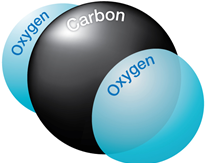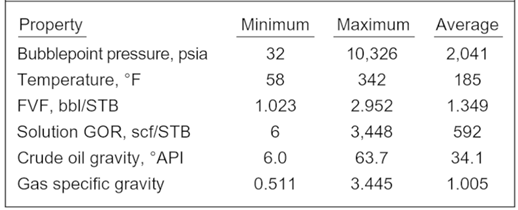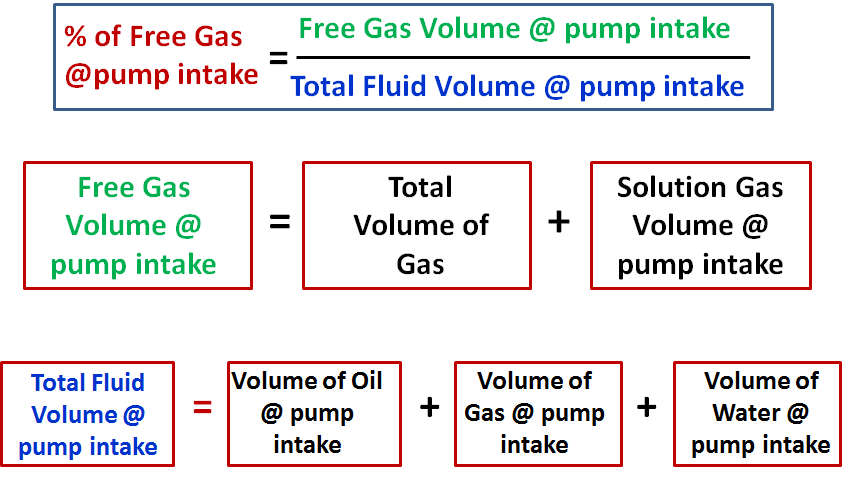## Effect of CO2 on downhole flowrate calculation

Downhole flow rate can be calculated from surface flow rate (stock tank barrels) using the following equation. It is assumed that no gas is dissolved in the water phase and the water formation volume factor is equal to one.

Downhole flow rate = [(Oil rate)sc × Bo] + [(Free GOR) × (Oil rate)sc × Gas FVF] + (Water rate)sc

Free GOR = Producing GOR – Solution GOR, therefore:

## q = ( Qo × Bo ) + [ ( R – Rs) × Qo × Bg × 1000] + Qw

Where:

• q = downhole flow rate (bbl/d or m3/d)
• Qo = Oil flow rate at standard conditions (stb/d or m3sc/d)
• Bo = Oil formation volume factor (bbl/stb or m3sc/m3sc)
• R = Producing gas-oil ratio (scf/stb or m3sc/m3sc)
• Rs = Solution gas-oil ration (scf/stb or m3sc/m3sc)
• Bg = Gas formation vol. factor (bbl/mscf or m3sc/m3sc)
• Qw = Water flow rate at standard conditions (stb/d or m3sc/d)

### Effect of CO2 on downhole flowrate calculation:If CO2 is present, the calculation of downhole flow rate becomes more complex for many reasons:

## PVT Properties and Correlations

Ideally, laboratory measured PVT data should be utilized. Many times, laboratory data is not available and correlations must be used instead. This post will discuss PVT properties and correlations that can be used to estimate them. It is difficult to say which correlation should be used when. This is because most of the correlations were developed with regional crude samples. The best correlation is the one that matches your data.Many investigators have used PVT laboratory test results, and field data, to develop generalized correlations for estimating properties of reservoir fluids. The main properties which are determined from empirical correlations are the bubble point, gas solubility, volume, density, compressibility, and viscosity. The correlations typically match the employed experimental data with an average deviation of less than a few percent. It is not unusual, however, to observe deviations with an order of magnitude higher when applied to other fluids.

1. Bubble Point Pressure (Pb):

The bubble point pressure, also known as the saturation pressure, is the pressure, at some reference temperature, that the first bubble of gas is liberated from the liquid phase. The reference temperature is usually the reservoir temperature, but any temperature can be used. Note that the bubble point pressure is a function of temperature and changing the reference temperature will change the bubble point pressure.

Statistical analysis of correlations:

Al-Shammasi, in his SPE paper “A Review of Bubble point Pressure and Oil Formation Volume Factor Correlations” (SPE-71302-PA, April 2001), compiled a databank of 1,243 data points from the literature. This was supplemented by 133 samples available from a GeoMark Research database (GeoMark Research. 2003. RFD base (Reservoir Fluid Database)), bringing the total number of data points to 1,376. These data were then used to rank the bubble point pressure correlations. The following Table summarizes the ranges of data used for bubble point pressure, temperature, oil FVF, Solution GOR, oil gravity, and gas specific gravity.## ESP design – Step 3: Gas Calculations

The presence of free gas in the tubing above the pump changes the fluid density, consequently reduces the required discharge pressure. Also the performance of centrifugal pumps is considerably affected by the presence of free gas in the pumped fluid. The pump starts producing lower than normal head as the produced GLR (Gas to Liquid Ratio) at the pumping conditions increases beyond a critical value. The critical value of the ratio or percentage of free gas present at the pump intake to the total volume of fluid depends on the pump impeller design (typical critical values are shown in the article “ESP: Gas handling device “). Therefore, it is essential to determine the percentage of free gas by volume at the pumping conditions in order select the proper pump and gas handling device (if required).

### Percentage of free gas by volume:

Assuming that Solution GOR (Rs), Gas Volume Factor (Bg) and Oil Formation Volume Factor (Bo) are known, the total volume of fluids and the percentage of free gas released at the pump intake should be calculated.